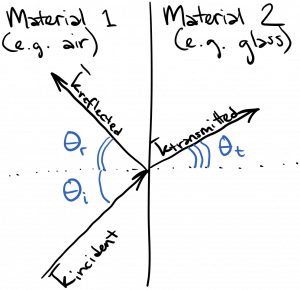Laser Photonics Light reflection and refraction: the basics

# Light reflection and refraction: the basics

I don’t like presenting facts without a derivation, but for the purposes of this chapter I’m going to just give you a little information about the reflection and refraction of plane waves at interfaces between two materials.  All of the relevant equations can be derived from a consideration of the electromagnetic boundary conditions, as can be seen in one of the many good textbooks on electromagnetics.

As you’re walking down the street on a sunny day, you pass a store window.  If you look in the window, you’ll probably see a reflection of yourself, and possibly some of what’s on the other side as well, depending on the properties of the glass and how brightly the room is lit.  So, we know that when light encounters an interface – like the interface between air and glass – it partly reflects, and partly transmits.  This is diagrammed below.I’ve drawn the wavevector for three waves: the incoming or incident wave, the reflected wave, and the refracted or transmitted wave.  Remember that the wavevector points in the direction that the wave is traveling (propagating).  We define the angles that each wavevector makes with respect to the normal to the surface separating material 1 from material 2.  Thus,$\theta_i$ is the incident angle,$\theta_r$ is the reflected angle, and$\theta_t$ is the transmitted angle.  You’ve likely seen the laws relating these angles; namely,$\displaystyle \theta_r = \theta_i$

i.e. the angle of reflection equals the angle of incidence, and$\displaystyle n_1 \sin{\theta_i} = n_2 sin{\theta_t}$

which is called Snell’s Law.$n_1$ is the refractive index of material 1, and$n_2$ is the refractive index of material 2.

It’s nice to know how all the angles are related, but this still doesn’t really tell us how much of the light is reflected and how much is transmitted.  Let’s consider the simplest case: light normally incident on a planar interface between two materials located at$z = 0$.  We can write the incident wave as$\displaystyle \vec{E}_{incident} = A_{i} \hat{a}_x \cos{(k_1 z - \omega t)}$

and then we can write the reflected wave as$\displaystyle \vec{E}_{reflected} = A_{r} \hat{a}_x \cos{(k_1 z + \omega t)}$

Notice that we have a plus sign inside the cosine of the reflected wave, as the wave is propagating in the$-z$ direction.  The amplitude of the incident wave is$A_{i}$ and the amplitude of the reflected wave is$A_{r}$.  The ratio$R = A_{r} / A_{i}$ is called the reflection coefficient.  For normal incidence$\theta_i = 0$, it turns out that$\displaystyle R = \frac{n_1 - n_2}{n_1 + n_2}$

I’m not deriving this equation right now, but it’s worthwhile to check if it makes any sense.  According to this, the reflection coefficient is zero if  material 1 and material 2 are the same material so that$n_1 = n_2$, which makes sense.  Also, the magnitude of the reflection coefficient increases as the difference between the refractive indecies increases, which also makes sense.  Please note that this equation is ONLY good for normal incidence!

We could also write the transmitted wave as$\displaystyle \vec{E}_{transmitted} = A_{t} \hat{a}_x \cos{(k_2 z - \omega t)}$

Note that this wave has a different wavenumber$k$ than the incident and reflected waves, because the wavenumber depends on the refractive index:$k = \omega n / c$.  The ratio$T = A_{t} / A_{i}$ is called the transmission coefficient, and for normal incidence$T = 1 + R$.

This equation might seem a little concerning – you probably have some instincts regarding conservation laws.  Indeed, power must be conserved.  The fraction of the incident power that is reflected from the interface is given by$|R|^2$, and the fraction of the incident power that is transmitted through the interface is given by$(n_2 / n_1) |T|^2$, and as you might expect, those fractions must add to 1:$\displaystyle |R|^2 + \frac{n_2}{n_1}|T|^2 = 1$